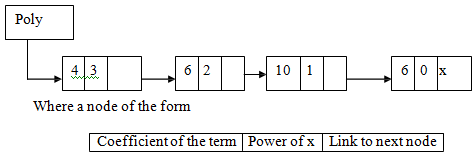## Usage of linked lists for polynomial manipulation, Data Structure & Algorithms

Assignment Help:

Q. Establish the usage of linked lists for polynomial manipulation.

Ans.

Usage of Linked List for Polynomial Manipulation.

Linked lists are frequently used for maintaining polynomial in memory. For

Example:

A polynomial of type 4x3 + 6x2 + 10x +6 can be represented using following linked list.#### Asymptotic notation, Asymptotic notation Let us describe a few function...

Asymptotic notation Let us describe a few functions in terms of above asymptotic notation. Example: f(n) = 3n 3 + 2n 2 + 4n + 3 = 3n 3 + 2n 2 + O (n), as 4n + 3 is of

#### Program on radix sort., Write a program that uses the radix sort to sort 10...

Write a program that uses the radix sort to sort 1000 random digits. Print the data before and after the sort. Each sort bucket should be a linked list. At the end of the sort, the

#### Explain dijkstra''s algorithm, Explain Dijkstra's algorithm Dijkstra's ...

Explain Dijkstra's algorithm Dijkstra's algorithm: This problem is concerned with finding the least cost path from an originating node in a weighted graph to a destination node

#### Two-dimensional array, Two-dimensional array is shown in memory in followin...

Two-dimensional array is shown in memory in following two ways:  1.  Row major representation: To achieve this linear representation, the first row of the array is stored in th

#### Frequency count, i:=1 while(i { x:=x+1; i:=i+1; }

i:=1 while(i { x:=x+1; i:=i+1; }

#### Write down a module to merge two linked lists, Two linked lists are having ...

Two linked lists are having information of the same type in ascending order. Write down a module to merge them to a single linked list that is sorted merge(struct node *p, stru

3633(mod 11)

#### Array implementation of a circular queue, A circular queue can be implement...

A circular queue can be implemented through arrays or linked lists. Program 6 gives the array implementation of any circular queue. Program 6: Array implementation of any Circu

#### Algorithm for linear search, Here,  m represents the unordered array of ele...

Here,  m represents the unordered array of elements n  represents number of elements in the array and el  represents the value to be searched in the list Sep 1: [Initialize]

#### Explain thread, Thread By changing the NULL lines in a binary tree to ...

Thread By changing the NULL lines in a binary tree to special links known as threads, it is possible to perform traversal, insertion and deletion without using either a stack